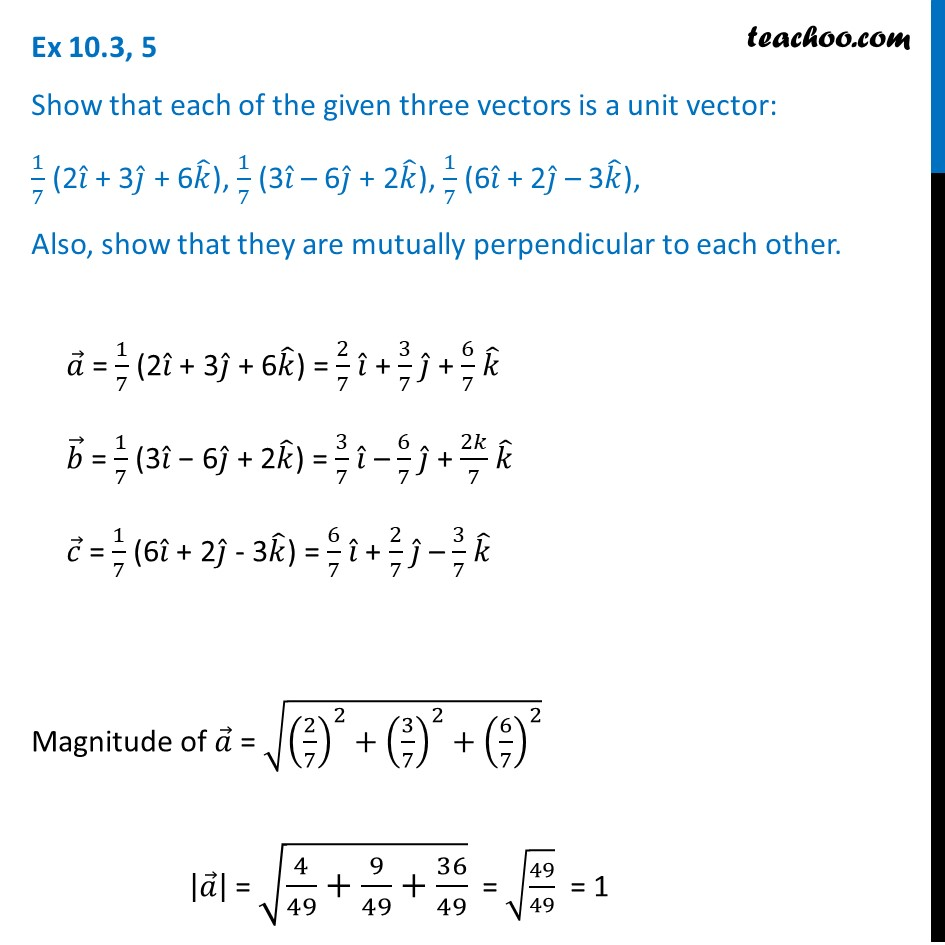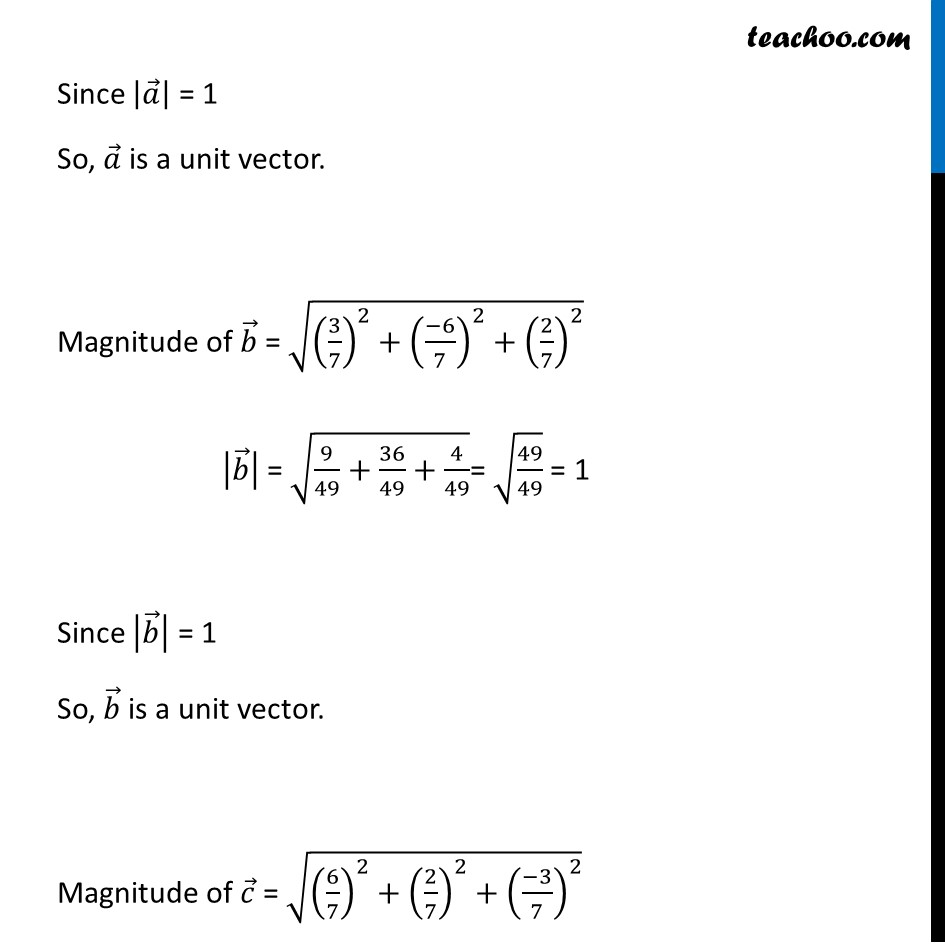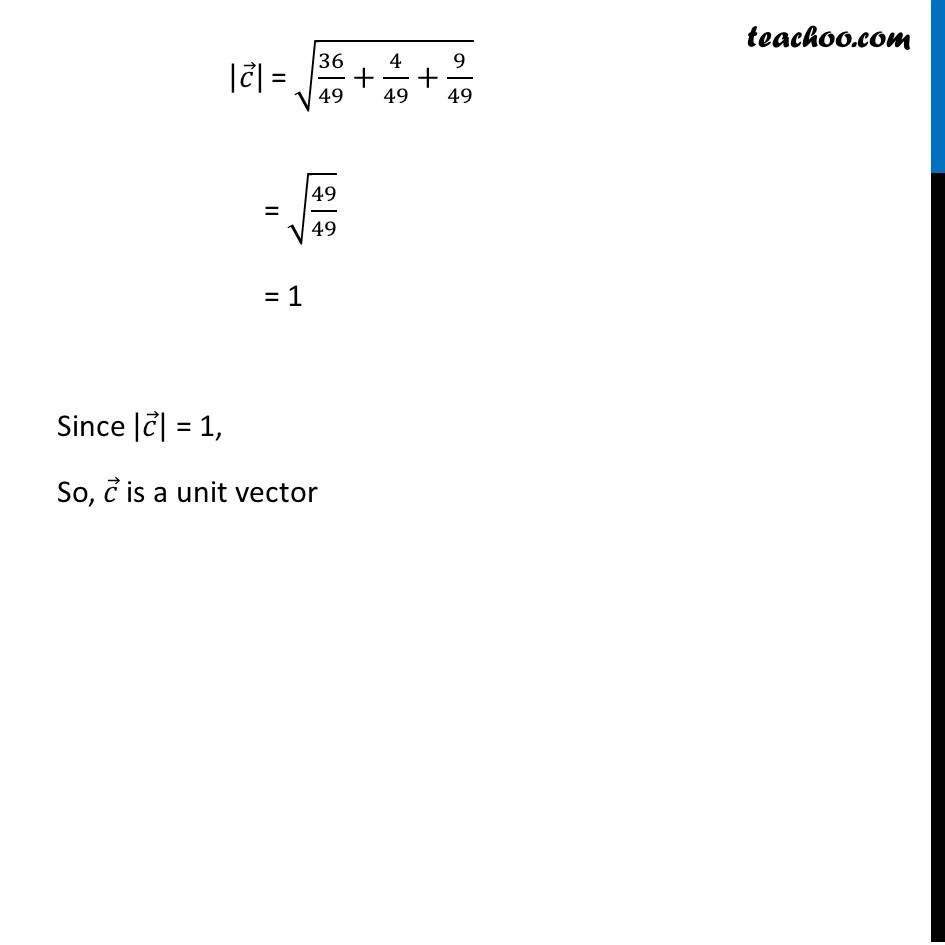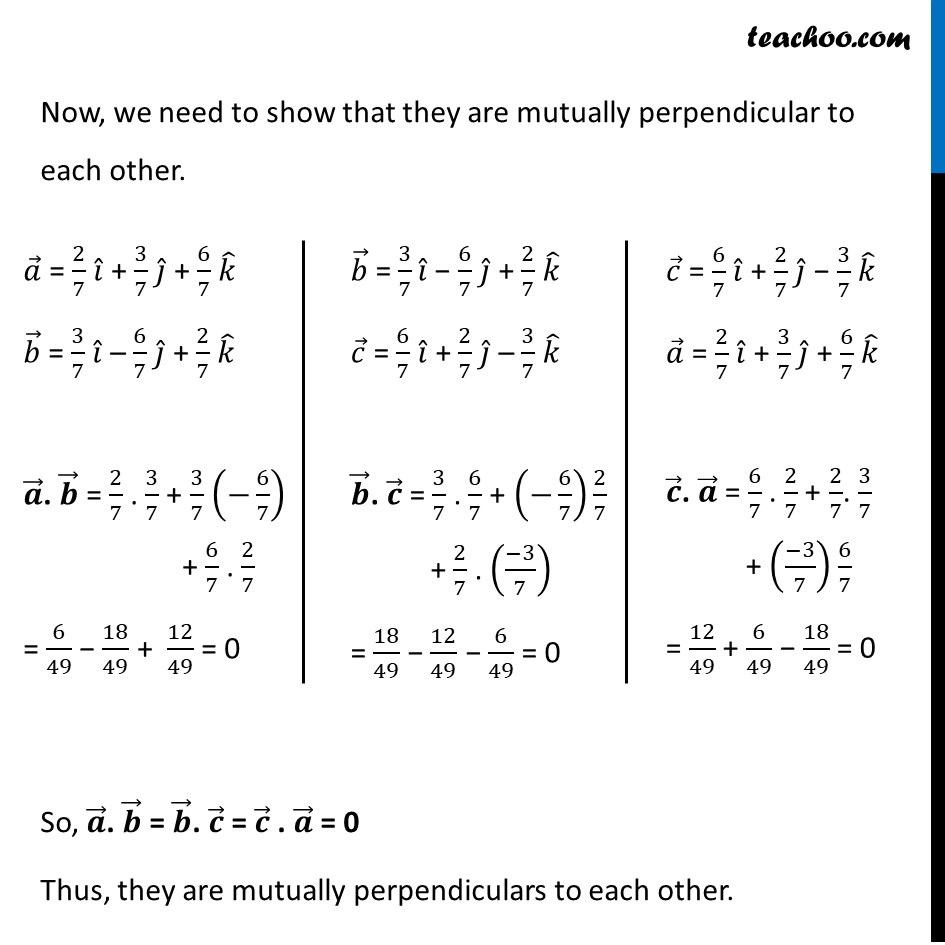Ex 10.3

Chapter 10 Class 12 Vector Algebra
Serial order wiseLearn in your speed, with individual attention - Teachoo Maths 1-on-1 Class

### Transcript

Ex 10.3, 5 Show that each of the given three vectors is a unit vector: 1/7 (2𝑖 ̂ + 3𝑗 ̂ + 6𝑘 ̂), 1/7 (3𝑖 ̂ – 6𝑗 ̂ + 2𝑘 ̂), 1/7 (6𝑖 ̂ + 2𝑗 ̂ – 3𝑘 ̂), Also, show that they are mutually perpendicular to each other. 𝑎 ⃗ = 1/7 (2𝑖 ̂ + 3𝑗 ̂ + 6𝑘 ̂) = 2/7 𝑖 ̂ + 3/7 𝑗 ̂ + 6/7 𝑘 ̂ 𝑏 ⃗ = 1/7 (3𝑖 ̂ − 6𝑗 ̂ + 2𝑘 ̂) = 3/7 𝑖 ̂ – 6/7 𝑗 ̂ + 2𝑘/7 𝑘 ̂ 𝑐 ⃗ = 1/7 (6𝑖 ̂ + 2𝑗 ̂ - 3𝑘 ̂) = 6/7 𝑖 ̂ + 2/7 𝑗 ̂ – 3/7 𝑘 ̂ Magnitude of 𝑎 ⃗ = √((2/7)^2+(3/7)^2+(6/7)^2 ) |𝑎 ⃗ | = √(4/49+9/49+36/49) = √(49/49) = 1 Since |𝑎 ⃗ | = 1 So, 𝑎 ⃗ is a unit vector. Magnitude of 𝑏 ⃗ = √((3/7)^2+((−6)/7)^2+(2/7)^2 ) |𝑏 ⃗ | = √(9/49+36/49+4/49)= √(49/49) = 1 Since |𝑏 ⃗ | = 1 So, 𝑏 ⃗ is a unit vector. Magnitude of 𝑐 ⃗ = √((6/7)^2+(2/7)^2+((−3)/7)^2 ) |𝑐 ⃗ | = √(36/49+4/49+9/49) = √(49/49) = 1 Since |𝑐 ⃗ | = 1, So, 𝑐 ⃗ is a unit vector Now, we need to show that they are mutually perpendicular to each other. So, 𝒂 ⃗. 𝒃 ⃗ = 𝒃 ⃗. 𝒄 ⃗ = 𝒄 ⃗ . 𝒂 ⃗ = 0 Thus, they are mutually perpendiculars to each other. 𝑎 ⃗ = 2/7 𝑖 ̂ + 3/7 𝑗 ̂ + 6/7 𝑘 ̂ 𝑏 ⃗ = 3/7 𝑖 ̂ – 6/7 𝑗 ̂ + 2/7 𝑘 ̂ 𝒂 ⃗. 𝒃 ⃗ = 2/7 . 3/7 + 3/7 (−6/7) + 6/7 . 2/7 = 6/49 − 18/49 + 12/49 = 0 𝑏 ⃗ = 3/7 𝑖 ̂ − 6/7 𝑗 ̂ + 2/7 𝑘 ̂ 𝑐 ⃗ = 6/7 𝑖 ̂ + 2/7 𝑗 ̂ – 3/7 𝑘 ̂ 𝒃 ⃗. 𝒄 ⃗ = 3/7 . 6/7 + (−6/7) 2/7 + 2/7 . ((−3)/7) = 18/49 − 12/49 − 6/49 = 0 𝑐 ⃗ = 6/7 𝑖 ̂ + 2/7 𝑗 ̂ − 3/7 𝑘 ̂ 𝑎 ⃗ = 2/7 𝑖 ̂ + 3/7 𝑗 ̂ + 6/7 𝑘 ̂ 𝒄 ⃗. 𝒂 ⃗ = 6/7 . 2/7 + 2/7. 3/7 + ((−3)/7) 6/7 = 12/49 + 6/49 − 18/49 = 0